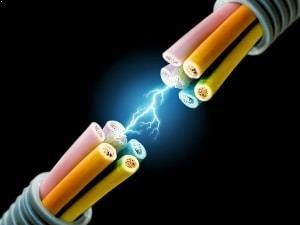# Car Audio Calculators

Most automotive consumers that are considering a car audio upgrade are just looking for a few new features with their car stereo or a little cleaner, crisper sound out of their door speakers. A true audiophile on the other hand, is looking for a complete audio system that will deliver crisp sound for every note and frequency.

Planning for a complete car audio project can be overwhelming when you start trying to calculate all of the factors that impact the acoustics of your car, the power your desire, and the components you plan on using. We put together these calculators to help you do some of the math required to build the car audio system of your dreams.VOLTAGE CALCULATOR

Voltage is the potential electrical difference, also referred to as electrical tension or electrical pressure, between two points which is equal to the electrical work that is done per unit of charge. Voltage or electrical potential is measured in volts and caused through electrical current through a magnetic field. Think of voltage as the man pushing the electronics through the wire, it represents the source of energy, referred to as electromotive force, which is lost, used, or stored. Automotive electrical systems run on a 12-volt direct current system to power everything from your ignition system to your car stereo and lighting.

This calculator is intended to help you calculate voltage levels of your car audio or lighting systems.

Instructions

Select two known electrical properties (Current/Power/Resistance), then enter those the two values and press "Calculate Voltage" button to get your answer. If you have more than one value to calculate, just change the value(s) you want to adjust and click the button again (if you change to a new measurement type - make sure you click the reset button).

Terms
E = Voltage (Volt)

I = Current (Amp)

P = Power (Watt)

R = Resistance (Ohm)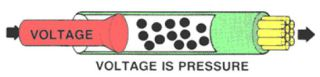##### Formulas

E = I x R

E = P / I

E = √ PXR

Amps
Watts
Ohms
VoltsIMPENDENCE / RESISTANCE CALCULATOR

Impendence is a measure of resistance in an electrical circuit which opposes the movement of electronics among atoms. The easier atoms accept or release electronics, the lower the resistance in your electrical circuit. Impendence is measured in ohms and is present in both alternating and direct current. Resistance in a circuit is largely related to the conductivity of the materials used in an electrical system. Materials such as copper, silver, and gold have low resistance which makes them great conductors of electricity. Materials such as rubber, mica, and glass have high resistance make them great insulators. Electrical wiring is made with a copper core with a low resistance to allow the electricity to flow and a rubber coating with its high resistance as an insulator.

This calculator is intended to help you calculate resistance/impendence levels of your car audio or lighting systems.

Terms

E = Voltage (Volt)

I = Current (Amp)

P = Power (Watt)

R = Resistance (Ohm)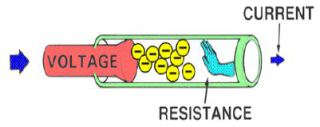Formulas

R = E / I

R = P / I2

E = E2/ P

Instructions

Select two known electrical properties (Current/Power/Voltage), then enter those the two values and press "Calculate Resistance" button to get your answer. If you have more than one value to calculate, just change the value(s) you want to adjust and click the button again (if you change to a new measurement type - make sure you click the reset button).

Volts
Amps
Watts
Ohms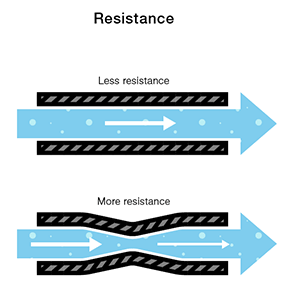POWER CALCULATOR

Power is a measurement of electrical energy which is transferred over time. Electrical power is measured in megawatts which is a measure of one million watts. A watt is an electrical unit which is generated or consumed. If you’re running a 100-watt amplifier, your amplifier is drawing 100 watts of electricity for every second its running.

This calculator is intended to help you calculate the power levels of your car audio or lighting systems.

Terms

E = Voltage (Volt)

I = Current (Amp)

P = Power (Watt)

R = Resistance (Ohm)Formulas
P = E / I

P = I 2/ R

P = E2 / R

Instructions

Select two known electrical properties (Current/Voltage/Resistance), then enter those the two values and press "Calculate Power" button to get your answer. If you have more than one value to calculate, just change the value(s) you want to adjust and click the button again (if you change to a new measurement type - make sure you click the reset button).

Volts
Amps
Ohms
Watts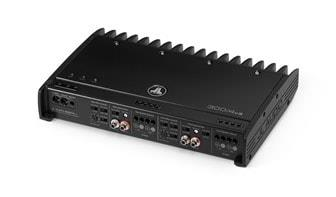CURRENT CALCULATOR

Current is a measure of the electrical flow, typically electrons or electron deficient atoms through a circuit. Current is measured in units called an ampere or amp for short which is identified by A. A simple ampere of current is represented as one coulomb of electrical charge which moves past a specific point in one second. Electrons, which are the most common electrical charge carriers, are negatively charged and flow from negative points to positive points (this of the positive and negative terminals on your car battery).

This calculator is intended to help you calculate current levels of your car audio or lighting systems.

Terms

E = Voltage (Volt)

I = Current (Amp)

P = Power (Watt)

R = Resistance (Ohm)Formulas
I = E / R

I = P / E

I =√P/R

Instructions

Select two known electrical properties (Power/Resistance/Voltage), then enter those the two values and press "Calculate Current" button to get your answer. If you have more than one value to calculate, just change the value(s) you want to adjust and click the button again (if you change to a new measurement type - make sure you click the reset button).

Volts
Watts
Ohms
Amps